# CBSE Solutions for Class 10 Maths

#### Select CBSE Solutions for class 10 Subject & Chapters Wise :

The angle of elevation from ground to the top of the building is 30° and the distance between the point and the base of the building is 10m. Find the height of the building.

Answer :

tanx = Height/Base

tan30° = Height/10

Height= 10/√3m

The angle of elevation from ground to the top of the building is 30° and the height of the building is 10/3m. Find the distance between the point and the base of the building.

Answer :

tanx = Height/Base

tan30° =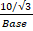Base = 10

The angle of elevation from ground to the top of the building is 60° and the distance between the point and the base of the building is 10m. Find the height of the building.

Answer :

tanx = Height/Base

Tan60° = Height/10

Height= 103m

If the distance between the base of the building and the point is 10m and the height of the building is 10/3m. Find the angle of elevation from ground to the top of the building.

Answer :

tanx = Height/Base

tanx=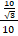= 1/3

x= 30°

The angle of elevation from ground to the top of the building is 60° and the height of the building is 103m. Find the distance between the point and the base of the building.

Answer :

tanx = Height/Base

tan60° =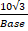Base = 10

If the distance between the base of the building and the point is 10m and the height of the building is 103m. Find the angle of elevation from ground to the top of the building.

Answer :

tanx = Height/Base

tanx=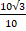= 3

x= 60°

The angle of elevation from ground to the top of the building is 60° and the height of the building is 5√3m. Find the distance between the point and the base of the building.

Answer :

tanx = Height/Base

tan60° =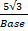Base = 5

The angle of elevation from ground to the top of the building is 30° and the distance between the point and the base of the building is 5m. Find the height of the building.

Answer :

tanx = Height/Base

tan30° = Height/5

Height= 5/3m

The angle of elevation from ground to the top of the building is 60° and the distance between the point and the base of the building is 5m. Find the height of the building.

Answer :

tanx = Height/Base

Tan60° = Height/5

Height= 5√3m

The angle of elevation from ground to the top of the building is 30° and the height of the building is 5/√3m. Find the distance between the point and the base of the building.

Answer :

tanx = Height/Base

tan30° =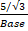Base = 5

A ladder 15 m long just reaches the top of a vertical wall. If the ladder makes an angle of 60° with the wall, then calculate the height of the wall. (2013OD)

Answer :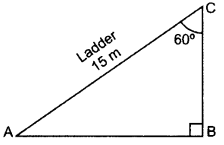BAC = 180° – 90° – 60° = 30°
sin 30° = BC/AC
1/2=BC/15
2BC = 15
BC = 15/2m

In the given figure, a tower AB is 20 m high and BC, its shadow on the ground, is 20√3m long. Find the Sun’s altitude. (2015OD)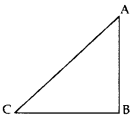Answer :

AB = 20 m, BC =20√3m,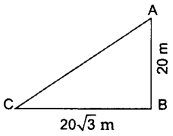In ∆ABC, Using trigonometry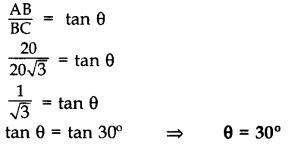​​​​​​​

In the figure, AB is a 6 m high pole and CD is a ladder inclined at an angle of 60° to the horizontal and reaches up to a point D of pole. If AD = 2.54 m. Find the length of the ladder. (Use √3 = 1.73) (2016D)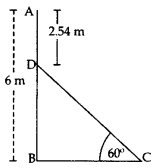Answer :

BD = AB – AD = 6 – 2.54 = 3.46 m
In rt., ∆DBC, sin 60° = BD/DC
√3/2=3.46/DC
√3 /DC = 3.46 x 2
Length of the ladder, DC = 6.92 /√3=6.92/1.73
DC = 4 m

A ladder, leaning against a wall, makes anangle of 60° with the horizontal. If the foot of the ladder is 2.5 m away from the wall, find the length of the ladder. (2016OD)

Answer :

Let us assume AC be the ladder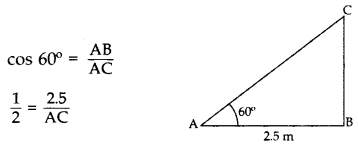Length of ladder, AC = 5 m 2.5 m

If a tower 30 m high, casts a shadow 10√3m long on the ground, then what is the angle of elevation of the sun? (2017OD)

Answer :

Let us assume required angle be θ.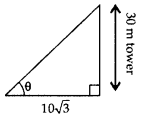tan θ = 30 /10 √3
tan θ = √3
tan θ = tan 60°

θ = 60°A=30° and AB=3cm

 1    ∠C A    2√3  cm 2    AC B    √3 cm 3    BC C    60° 4    ∠B D    90°

Answer :

1-C, 2-A, 3-B, 4-DC=60° and BC= 3 cm

 1 ∠A A 2√3  cm 2 AB B 30° 3 AC C 90° 4 ∠B D 3cm

Answer :

1-B, 2-D, 3-A, 4-CC=45° and BC= 3 cm

 1 ∠A A 90° 2 AB B 45° 3 AC C 3 cm 4 ∠B D √6

Answer :

1-B, 2-C, 3-D, 4-A

### Take a Test

Choose your Test :

### Chapter 9 : Some Applications of Trigonometry

In this chapter, “ Some applications of trigonometry”, class 10 students will get to know how trigonometry is helpful in finding the height and distance of different objects without measuring. In earlier days, astronomers have used trigonometry for calculating the distance from the earth to the planets and stars. Trigonometry is mostly used in navigation and geography to locate the position in relation to the latitude and longitude.

### Browse & Download CBSE Books For Class 10 - All Subjects

The GSEB Books for class 10 are designed as per the syllabus followed Gujarat Secondary and Higher Secondary Education Board provides key detailed, and a through solutions to all the questions relating to the GSEB textbooks.

The purpose is to provide help to the students with their homework, preparing for the examinations and personal learning. These books are very helpful for the preparation of examination.

For more details about the GSEB books for Class 10, you can access the PDF which is as in the above given links for the same.